Thermos Index

Thermodynamics Cycles

Introduction

Various internal combustion engine types have been devised and represented by various idealised cycles (otto cycle for four stroke, diesel cycle etc.. These idealised cycles are useful for determining the practical limitations and efficiencies possible.  They do not however provide the answer to the question..

"What is the greatest fraction of the heat transfer from a energy source is it possible to convert into work ? i.e. what is the limiting efficiency of conversion ?"

Carnot introduced a theoretical gas cycle based on ideal reversible process which provides this information

Carnot Cycle

Carnot in 1824 arrived at the "carnot cycle" which is an idealised gas cycle that obtains the maximum amount of work from an engine working in a thermodynamically reversible manner.  This cycle provides a maximum efficiency for any thermodynamic heat engine

The Carnot cycle for perfect gases is an idealised cycle composed of four reversible processes

 an isothermal expansion of fixed mass of gas (say in a cylinder- causing a piston to move out) an adiabetic expansion the gas (say in a cylinder- causing a piston to move out) an isothermal contraction of the gas (say in a cylinder- causing a piston to move in) an adiabetic contraction of the gas (say in a cylinder- causing a piston to move in)

This imaginary working fluid is contained in the (cylinder)closed system and simply receives and rejects energy to a source and sink using perfect heat transfer (with no temperature difference ).  As a result of receiving and rejecting energy it expands and contracts during four ideal reversible "no-flow" processes.  The fluid is an ideal gas following the ideal gas laws.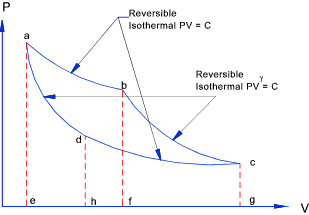The work done through during a complete cycle is determined using the relationships identified on webpage Polytropic processes....

From the general relationship for adiabatic polytropic processes the following relationship is identified. Relationships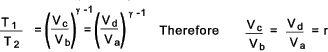Reversible Process Heat Transfer at T1 to Working Fluid From Hot Source Heat Rejected at T2 from Working Fluid From Sink Work done by working fluid Change in Internal Energy of Fluid Isothermal Expansion RmT1loger 0 RmT1loger 0 Adiabatic Expansion 0 0 Rm(T1 - T2 )/ (1- γ ) -Rm(T1 - T2 )/ (1- γ ) Isothermal Compression 0 RmT2loger -RmT2loger 0 Adiabatic Compression 0 0 -Rm(T1 - T2 )/ (1- γ ) Rm(T1 - T2 )/ (1- γ ) Totals RmT1loger = Q1 RmT2loger = Q2 Rm(T1- T2 )loger= W 0

From the table it can clearly be seen that the total work done by the carnot cycle is Rm(T1 - T1 )loge r = Q1 - Q2.

The energy supplied = RmT1 loge r = Q1. Therefore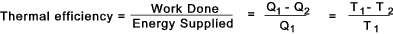This is the maximum efficiency achievable by an reversible thermodynamic cycle working with a ideal perfect gas.

The following relationship results from the above....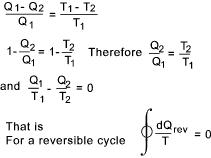Air Standard cycles

Although the Carnot cycle is theoretically the most efficient it is in no way a practical device.   Also the energy transfers would be far too slow for any real benefits to be realised.    Internal combustion engines work on non cyclic processes because the fuel-air mix enters the system and products of combustion exit the system.  .  However theoretical cycles based on the hypothesis that air is the working fluid in a closed system receiving an rejecting energy to external sinks allows provide very crude estimations on the theoretical efficiencies possible internal combustion engines.

For the purpose of the air standard cycles the suction and exhaust strokes are not considered.T

The Otto Cycle or constant volume cycle has been proposed to provide an approximation of the 4 stroke Internal combustion cycle designed by Otto.  The diesel cycle is used to approximate a cycle with heat being added at constant pressure..

Otto Cycle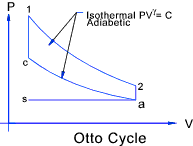The Otto cycle is comprised of four reversible processes of air in a closed system:

 a -> c adiabatic compression, c -> 1 constant volume heat addition 1 -> 2adiabatic expansion, 2 -> a and constant volume heat rejection

The diagram for this process is shown above.

The compression ratio r = V2 / V1

Heat is added (Q1) between c and 1 = mcv(T1 - Tc) and Heat is rejected (Q2 )between 2 and b= mcv(T2 - Ta)
There is no heat added or rejected during the adiabatic expansion or compression therefore the thermal efficiency of this ideal process is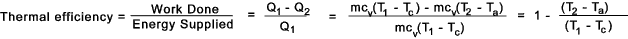This expression can be simplified using the following adiabatic relationship Relationships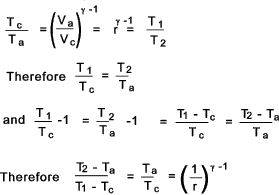The thermal efficiency of and otto cycle is therefore.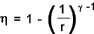Diesel Cycle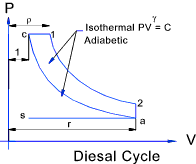This hypothetical cycle is one with heat being rejected at constant pressure. The stages of the cycle are shown below.

 c - > 1 Energy is added by heat transfer at constant pressure 1 - >2 Adiabatic expansion 2 - > a Energy rejected by heat transfer at constant volume a - > c Adiabatic compression

Energy supplied during c-1 = mcp (T1 - Tc )
Energy rejected during 2-a = mcv (T2 - Ta )
The thermal efficiency of the cycle =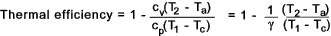This can be simplified as follows: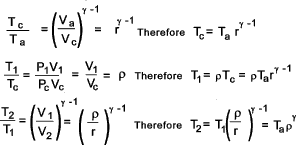The Thermal efficiency is now simplified as follows...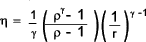Air Cycle Links Thermodynamics..NASA - Glenn Research center at Series of informative notes on Thermodynamics thermodynamicstudy The carnot cylces..Interesting Article Clausius inequality..A very nice page on the Clausius Inequality

Thermos Index